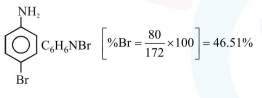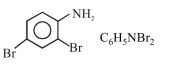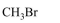# In Carius method of estimation of halogen,

Question:

In Carius method of estimation of halogen, $0.172 \mathrm{~g}$ of an organic compound showed presence of $0.08 \mathrm{~g}$ of bromine. Which of these is the correct structure of the compound :

1.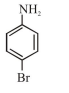2.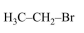3.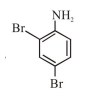4.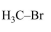Correct Option: 1

Solution:

In Carius method

mass of organic compound $=0.172 \mathrm{gm}$

mass of Bromine $=0.08 \mathrm{gm}$

Hence $\%$ of Bromine $=\frac{0.08}{0.172} \times 100$

$=46.51 \%$